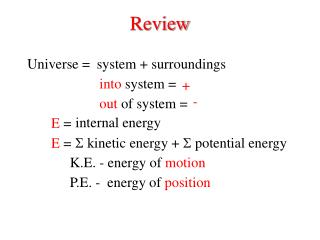Download PresentationReviewReview - PowerPoint PPT Presentation

Download PresentationReview
An Image/Link below is provided (as is) to download presentation

Download Policy: Content on the Website is provided to you AS IS for your information and personal use and may not be sold / licensed / shared on other websites without getting consent from its author. While downloading, if for some reason you are not able to download a presentation, the publisher may have deleted the file from their server.

- - - - - - - - - - - - - - - - - - - - - - - - - - - E N D - - - - - - - - - - - - - - - - - - - - - - - - - - -
Presentation Transcript

1. Review • system + surroundings Universe = • into system = • + • - • out of system = internal energy E =  kinetic energy • +  potential energy E = • K.E. - energy of motion P.E. - energy of position

2. Euniverse = 1st Law of Thermodynamics Energy can not be created or destroyed Euniverse= 0 Esystem + Esurroundings = 0 - Esurroundings Esystem = heat = q Energy is exchanged as : work = w E = q + w

3. heat flow of energy along a T gradient If T1 > T2 System at T1 Surroundings at T2 a) > b) < c) = • q system • 0 Exothermic reaction • q surroundings • > 0

4. heat flow of energy along a T gradient If T1 < T2 System at T1 Surroundings at T2 > 0 • q system Endothermic reaction • q surroundings • < 0

5. work Electrical work Mechanical work = force x distance force = pressure x area = P x m2 distance = m work = = P x V P x m2 x m Pext V - Wsystem=

6. State Functions Property that depends only on the initial and final states Temperature, T : raise T from 298  300 K path 1 T = Tfinal - Tinitial= 300 - 298 = 2 K : raise T 298  500 K path 2 lower T from 500  300 K T = Tfinal - Tinitial = 300 - 298 = 2 K

7. Extensive v.s. Intensive Extensive: Proportional to the mass of the system Intensive: Independent of the mass of the system Temperature: Intensive or Extensive Volume : Intensive or Extensive Pressure: Intensive or Extensive Internal Energy: Intensive or Extensive

8. System 1 How much work will be done as the gas expands against the piston? Pext = 1.5 atm, T = 298 K P1 = 6.0 atm V1 = 0.4 L V2 = 1.6 L P2 = 1.5 atm P1 V1 = P2 V2 PV = nRT (6.0 atm) (0.4 L) = (1.5 atm) (V2) w = -PextV -(1.5 atm) -1.8 L atm w = (1.6 - 0.4)L = (-1.8 L atm) (101.3 J/L atm) = -182 J

9. System 2 How much work is done when the stopcock is opened? ideal gas vacuum 0.4 L 1.2 L 6.0 atm P1 = 6 atm P2 = 1.5 atm V1 = 0.4 L V2 = 1.2 + 0.4 L = 1.6 L T1 = 298 K = T2 P1 V1 = P2 V2 w = -PextV = -(0 atm) (1.6 L - 0.4 L) w = 0

10. System 1 System 2 P1 = P2 = V1 = V2 = T1 = T2 = 6.0 atm 1.5 atm P1 = P2 = V1 = V2 = T1 = T2 = 6.0 atm 1.5 atm 0.4 L 1.6 L 0.4 L 1.6 L 298 K 298 K w = -182 J q = +182 J w = 0 q = 0 Same initial and final conditions  all State functions are the same E will be the same if T = 0, E = 0 Ideal gases : E = q + w = 0

11. Thermochemistry State 1 = State 2 = reactants products E = Eproducts - Ereactants Endothermicreaction  2NH3(g) + Ba(SCN)2(l) + 10H2O(l) + 2NH4SCN (s) Ba(OH)2•8H2O (s) a) < b) > c) = a) < b) > c) = q 0 w 0 reaction lowered T of system

12. EndothermicReaction Tprod< Treact K.E.prod < K.E.react P.E.prod> P.E.react productsless stable than reactants

13. Exothermic Reaction Tprod> Treact K.E.prod > K.E.react P.E.prod< P.E.react  mix of gases C12H24O12 (s) + KClO3(s) q 0 < w 0 < products more stable than reactants